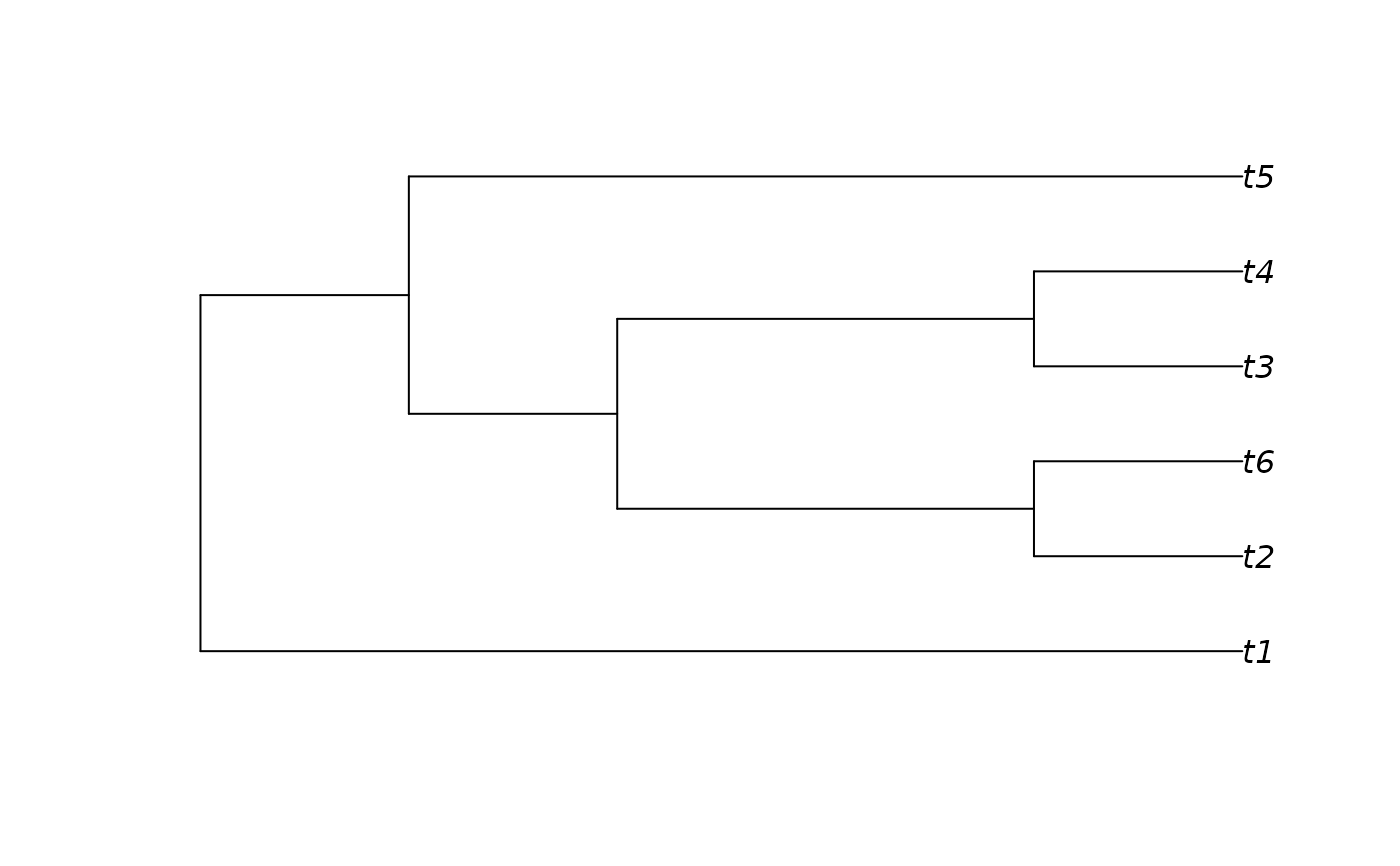Functions converting between phylogenetic trees and their unique decimal representation, based on a concept by John Tromp, employed in (Li et al. 1996) .

as.TreeNumber(x, ...)

# S3 method for phylo
as.TreeNumber(x, ...)

# S3 method for multiPhylo
as.TreeNumber(x, ...)

# S3 method for character
as.TreeNumber(x, nTip, tipLabels = TipLabels(nTip), ...)

# S3 method for TreeNumber
as.TreeNumber(x, ...)

# S3 method for MixedBase
as.TreeNumber(x, ...)

# S3 method for TreeNumber
as.MixedBase(x, ...)

# S3 method for integer64
as.MixedBase(x, tipLabels = NULL, ...)

# S3 method for numeric
as.MixedBase(x, tipLabels = NULL, ...)

# S3 method for numeric
as.phylo(x, nTip = attr(x, "nTip"), tipLabels = attr(x, "tip.label"), ...)

# S3 method for TreeNumber
as.phylo(x, nTip = attr(x, "nTip"), tipLabels = attr(x, "tip.label"), ...)

as.MixedBase(x, ...)

# S3 method for MixedBase
as.MixedBase(x, ...)

# S3 method for phylo
as.MixedBase(x, ...)

# S3 method for multiPhylo
as.MixedBase(x, ...)

# S3 method for MixedBase
as.phylo(x, nTip = attr(x, "nTip"), tipLabels = attr(x, "tip.label"), ...)

## Arguments

x

Integer identifying the tree (see details).

...

Additional parameters for consistency with S3 methods (unused).

nTip

Integer specifying number of leaves in the tree.

tipLabels

Character vector listing the labels assigned to each tip in a tree, perhaps obtained using TipLabels().

## Value

as.TreeNumber() returns an object of class TreeNumber, which comprises a numeric vector, whose elements represent successive nine-digit chunks of the decimal integer corresponding to the tree topology (in big endian order). The TreeNumber object has attributes nTip and tip.label.

as.phylo.numeric() returns a tree of class phylo.

## Details

There are NUnrooted(n) unrooted trees with n leaves. As such, each n-leaf tree can be uniquely identified by a non-negative integer x < NUnrooted(n).

This integer can be converted by a tree by treating it as a mixed-base number, with bases 1, 3, 5, 7, … (2 n - 5).

Each digit of this mixed base number corresponds to a leaf, and determines the location on a growing tree to which that leaf should be added.

We start with a two-leaf tree, and treat 0 as the origin of the tree.


0 ---- 1


We add leaf 2 by breaking an edge and inserting a node (numbered 2 + nTip - 1). In this example, we'll work up to a six-leaf tree; this node will be numbered 2 + 6 - 1 = 7. There is only one edge on which leaf 2 can be added. Let's add node 7 and leaf 2:


0 ---- 7 ---- 1
|
|
2


There are now three edges on which leaf 3 can be added. Our options are:

Option 0: the edge leading to 1;

Option 1: the edge leading to 2;

Option 2: the edge leading to 7.

If we select option 1, we produce:


0 ---- 7 ---- 1
|
|
8 ---- 2
|
|
3


1 is now the final digit of our mixed-base number.

There are five places to add leaf 4:

Option 0: the edge leading to 1;

Option 1: the edge leading to 2;

Option 2: the edge leading to 3;

Option 3: the edge leading to 7;

Option 4: the edge leading to 8.

If we chose option 3, then 3 would be the penultimate digit of our mixed-base number.

If we chose option 0 for the next two additions, we could specify this tree with the mixed-base number 0021. We can convert this into decimal:

0 × (1 × 3 × 5 × 9) +

0 × (1 × 3 × 5) +

3 × (1 × 3) +

1 × (1)

= 10

Note that the hyperexponential nature of tree space means that there are > 2^64 unique 20-leaf trees. As a TreeNumber is a 64-bit integer, only trees with at most 19 leaves can be accommodated.

Describe the shape of a tree topology, independent of leaf labels: TreeShape()

Other tree generation functions: ConstrainedNJ(), GenerateTree, NJTree(), TrivialTree

## Author

Martin R. Smith (martin.smith@durham.ac.uk)

## Examples

tree <- as.phylo(10, nTip = 6)
plot(tree)as.TreeNumber(tree)
#> Phylogenetic tree number 10 of 105
#>  6 tips: t1 t2 t3 t4 t5 t6

# Larger trees:
as.TreeNumber(BalancedTree(19))
#> Phylogenetic tree number 3259279213732796827 of 6332659870762850625
#>  19 tips: t1 t2 t3 t4 t5 t6 t7 t8 t9 t10 t11 t12 t13 t14 t15 t16 t17 t18 t19

# If > 9 digits, represent the tree number as a string.
treeNumber <- as.TreeNumber("1234567890123", nTip = 14)
tree <- as.phylo(treeNumber)
as.phylo(0:2, nTip = 6, tipLabels = letters[1:6])
#> 3 phylogenetic trees